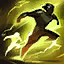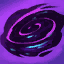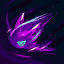League of Legends
LoL# Vex·KombaMage

·

Average

Power Spike

 Stupeň S- Win rate 52.8%Pick rate 7.6%Ban rate 10.8%RFRWAAEQAA

Start with R then flash in then R then W then AA then E then Q then finish with AA

Zvláštní článek
Hard
Zvláštní článekEQRRW

Start with E then Q then use R twice then finish with W

AverageFWERQRAA

Flash in then W then E the R the Q then R then AA

AverageRRWEQAA

Start with R then R again then W then E then Q then finish with AA

AverageERQAARAAWAA

Start with E then R then Q then AA then R then AA then W then finish with AA

AverageAAEAAQAA

EasyAAWQEAA

Start with AA then W then Q then E then finish with AA

EasyFiltersSections